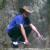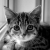awk 分割字符串，但是我只要以第一个符号分割

【推荐阅读】滴滴出行的数据架构和信息流处理思路解析->>>sql1=select name,age frome peop_info where 1=1

\$awk -F "=" '{print \$2}' 这样遇到有多个等号的就只取第一个等号后面，第二个等号前面的

0

~\$ echo "sql1=select name,age frome peop_info where 1=1" | awk -F'=' '{s="";for(i=2;i<=NF;i++)s=s""(i==NF?\$i:\$i"=");print s}'

select name,age frome peop_info where 1=1
0localhost:~ macbook\$ echo "sql1=select name,age frome peop_info where 1=1" | cut -d '=' -f 2-
select name,age frome peop_info where 1=1
localhost:~ macbook\$

0t="sql1=select name,age frome peop_info where 1=1"

echo \${t/sql1=/}